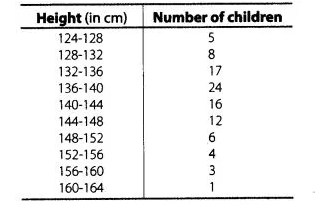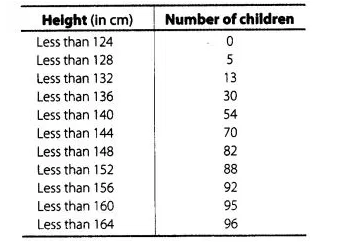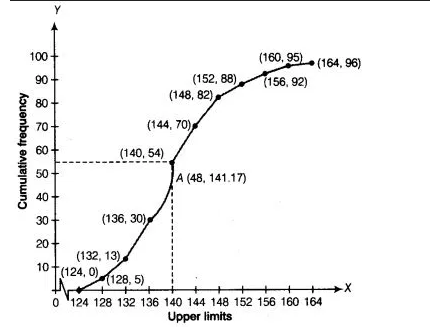# The distribution of heights (in cm) of 96

Question:

The distribution of heights (in cm) of 96 children is given belowDraw a less than type cumulative frequency curve for this data and use it to compute median height of the children.

Solution:To draw the less than type ogive, we plot the points (124, 0), (128, 5), (132, 13), (136, 30), (140, 54), (144, 70), (148, 82), (152, 88), (156, 92),

(160, 95), (164, 96) and join all these point by free hand.Here, $\frac{N}{2}=\frac{96}{2}$

We take, y = 48 in Y-coordinate and draw a line parallel to X-axis, meets the curve at A and draw a perpendicular line from point A to the X-axis

and this line meets the X-axis at the point which is the median i.e., median = 141.17.